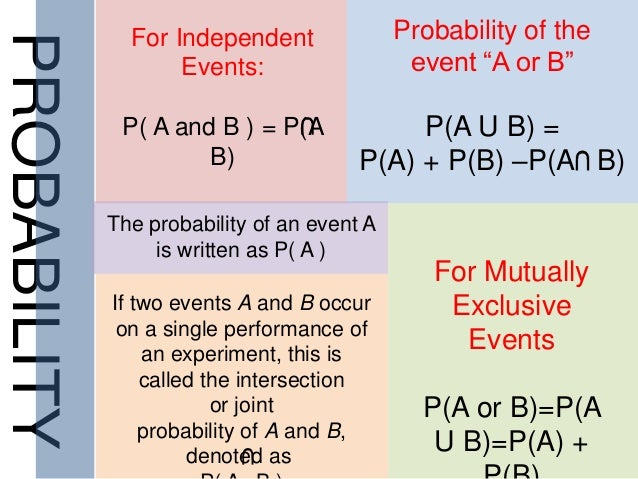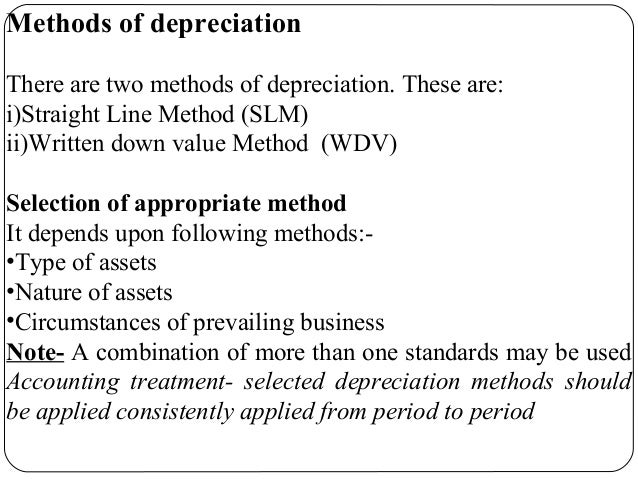Hola ! It's been a long while since I posted here. Well, first we had Dussehra / Gandhi Jayanti long weekend, hence there were no classes. So we guys met after a break of about 2 weeks. If you remember, before the long break, we were told to be prepared for a quiz in Financial Accounting - last post. So here's how Day 8 - Executive PGDM at NMIMS, Hyderabad was.

## Probability Lessons

The day started with Statistics. Till now we had studied about the basic fundas including Mean, Median, Mode, Variance, Standard Deviation. Last class we learnt about Probability and today we built upon the same. Today was a pretty good class where we learnt about assigning probabilities for events that have no fixed outcome. For Eg.: a case of project management where you need to mention how much time you will take to complete. In that case, the project can take say anywhere between 8 months to 12 months. But to predict the time needed, we need historic data based on which we can assign the relative frequencies. It's not like tossing a coin where we can simply assign 1/2 to {H} and  {T}. So yeah, solved a couple of examples. Good class.Probability

## Accounting Quiz

Next session was Accounting and the entire class was discussing about clearing it. The quiz was supposed to be for 5 marks (yes, the only 5 mark test I've ever given till date) Anyways, since the paper was still being printed, we first spent time on the next chapter. We learnt about Long Lived Assets and who depreciation affects the accounts. We learn the various methods of calculating depreciation:

1. Straight Line Method: here the cost of the item is distributed equally over the life time of the equipment. Say you bought a computer for Rs 5000 and it's lifetime is supposed to be 5 years, then the depreciation would be 5000/5 = Rs1000 per year. This is an easy way to calculate the depreciation, however it doesn't assign the depreciation cost in the correct manner.
2. Written Down Value Method: this is a more practical approach where in first the depreciation value for the first year is calculated and the cost value is found. From next year onward, the depreciation value will be based on the cost value and thus the depreciation value keeps on decreasing over the period of time.
3. Sum of Years Method: In this case, we take into account the cost value when the total cost and the residual values are provided. We use the formula [k/s x Residual Cost]. Here k is the year and S is the sum of all the years.
4. Units of Production: For this we are provided how many items are produced by the company each year. We then calculate a price value using (residual value / units produced) and multiply it by the Price value to calculate depreciation for every year.Ways to calculate Depreciation

Of all the methods, the most widely used are Written Down Value and Straight Line Method. Also one thing to note is that Indian authorities have allowed the companies to use any of the method. Using the Written Down Value, the depreciation value increases and thus the tax reduces. However to the investors they show the same value using Straight Line Method. Concept wise it was heavy, so need to spend time.

Anyways, coming to the Accounting Quiz, it was pretty good. The questions were of a decent toughness level as anyone who understood the basic concepts would have cleared. The faculty was way too quick to correct the papers, by evening we already got the marks. Well, I'm happy with the marks ! I won't say it here, but it was pretty good :)

## Speeding through ITM

The last lecture of the day was IT for Managers. We started by learning about Databases and DBMS. Introduced to the basics of DBMS, DML, DDL and the likes. We were reminded about EF Codd rules that I learnt during my engineering days. Also there were few examples about SQL queries. Post this we zoomed through SDLC methods including Waterfall, V-Process, Rapid, Spiral, Agile and the likes. Frankly, for me it was pretty easy but I wonder how other folks from non computer backgrounds will handle this. Well I guess, just like how I am struggling with Accounts. !Why Requirement Gathering is important !

Overall it was an eventful day Day 8 - Executive PGDM at NMIMS, Hyderabad. Next week we have a double doze  of classes so Saturday and Sunday are going to busy. So stay tuned, till then do what you love ! PS: All that I talk here about the subjects are solely based on my recollection. I'm not having a book with me while writing the post so the things 'might' be erroneous.
Day 8 - Executive PGDM at NMIMS, HyderabadReviewed by The Executive on October 08, 2017 Rating: 5